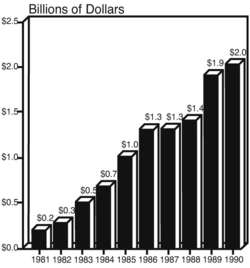bar chart

(redirected from bar graphs)
Also found in: Dictionary, Thesaurus, Medical, Financial.

bar chart

[′bär ‚chärt]
(statistics)Fig. 2 Bar Chart. A diagrammatic display of a frequency distribution in which bars of equal width represent each category, with the length of each bar being proportional to the number or frequency of occurrence for each category. In the above example, the annual student loan default in \$US is represented for the years 1981-1990.

bar chart

a diagrammatic method for displaying FREQUENCY DISTRIBUTIONS, in which bars of equal width are drawn to represent each category, with the length of each bar being proportional to the number, or frequency of occurrence, of each category (see Fig. 2). Bar charts are used not because they present different information than can be displayed in tables, but because they can display it in a way that is easier to understand. See also HISTOGRAM.

bar chart

A graphical representation of information in the form of bars. See business graphics.
References in periodicals archive ?
This argument reiterates the earlier point that bar graphs are effective for presentations but are not always so for scientific papers.
At the end of the second week in October, we review the parts of a bar graph then students construct their graph of our collected weather data to keep in their mathematics logs.
The line graph showed continuous curves, the bar graphs and pictograms depicted nine data points, and the tables showed either 6 or 15 data points.
For example, check out the sequence of diagrams that accompanies the bar graph below.
We'll show you how to make two: a bar graph and a line graph.

Site: Follow: Share:
Open / Close